# Dual functions

Functions complementary in the sense of Young, i.e. strictly convex functions (cf. Convex function (of a real variable)) connected by the Legendre transform.

For certain real-valued non-decreasing functions defined on the positive half-line (including zero) there is a natural notion of an inverse. Ifandare such inverses to each other, the functionsand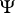defined (on the positive half-line) by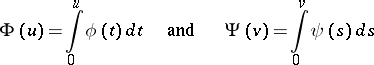are said to be complementary in the sense of Young or Young-conjugate. For them Young's inequality holds: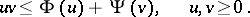Associated with a pair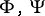of non-vanishing functions complementary in the sense of Young and a-finite measure, there is a pair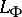,of complete normed spaces. These spaces, consisting of (equivalence classes of)-measurable functions, are called Orlicz spaces (cf. Orlicz space). The Lebesgue spaces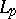(cf. Lebesgue space) are particular cases of Orlicz spaces, cf. [a4].

In a more abstract setting, the name dual functions is reminiscent of dual pair in duality theory and of dual problems in convex programming and optimal control (cf. Optimal control, mathematical theory of), but this name is rarely used in English: the most common name is (convex) conjugate functions (cf. Conjugate function).

Letandbe two real vector spaces in separate duality with respect to a bilinear form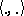(the usual one if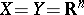), and letbe a mapping frominto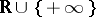(ifis only defined on a subsetof, seton, the complement of). If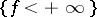is non-empty, the dual, or polar, or adjoint, or better conjugate function ofis the convex function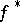defined onby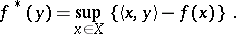The following result is a generalization of the geometric Hahn–Banach theorem on the bipolar of a set: the biconjugate function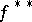ofis the greatest lower semi-continuous convex function bounded above by, and so is equal toif and only ifis a lower semi-continuous convex function (in which case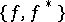is called a pair of conjugate functions). The notion of conjugate function, which was introduced by W. Young in the case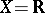and by W. Fenchel in the case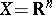, is very important in convex analysis; it is closely related to the notion of subdifferential: ifis convex and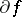is its subdifferential, then for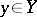andone has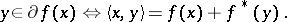This can be written, ifis lower semi-continuous, asThe mapping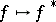is often called the Fenchel transform, sometimes with the name of Young or Legendre, or both, added. Whenandis sufficiently smooth as a convex function, it is a special case of the Legendre transform; on the other hand, it is also a special case of a Galois correspondence; these facts are of secondary importance in convex analysis. The notion of conjugate function plays a fundamental role in convex optimization. It is used to define the Lagrangian of some problem and the associated dual problem.

When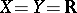, a function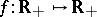is called a Young function if it is a non-decreasing convex function such that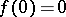and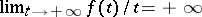. The conjugate functionof a Young functionis still a Young function on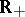; for example, when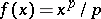with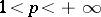, thenwhereis the conjugate exponent of, i.e.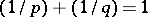. Young functions are used to define Orlicz spaces (cf. Orlicz space), and pairs of conjugate Young functions are used to study the duality between them; more generally they help to establish various inequalities in measure theory (Burkholder inequalities in martingale theory, Chernov's inequality in classical probability theory, Kullback's inequality in statistics, etc.), via the easy to prove but fundamental Young inequality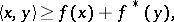which enabled Young to solve a problem about Fourier transformation.